# Axis

15 May 201724 minutes to read

Charts typically have two axes that are used to measure and categorize data: a vertical (y) axis, and a horizontal (x) axis.

Vertical axis always uses numerical or logarithmic scale. Horizontal(x) axis supports the following types of scale:

• Category
• Numeric
• DateTime
• DateTime Category
• Logarithmic

## Category Axis

Category axis displays the text labels instead of numbers. To use the categorical axis, you can set the `valueType` property of the axis to the category. Default value of `valueType` is double.

• js
• ``````"use strict";
var primaryXAxis={
//Use categorical scale in primary X axis
valueType: 'category',
};

ReactDOM.render(
<EJ.Chart id="default_chart_sample_0"
primaryXAxis= {primaryXAxis}>

</EJ.Chart>,
document.getElementById('chart')
);``````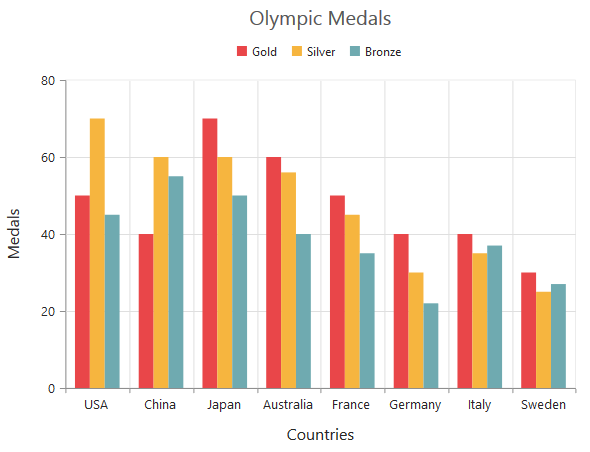Click here to view our online demo sample that uses Category axis.

### Place labels on ticks

Labels in the category axis can be placed on the ticks by setting the `labelPlacement` property of axis to the onticks. The default value of the `labelPlacement` property is betweenticks i.e. labels are placed between the ticks, by default.

• javascript
• ``````"use strict";
var primaryXAxis={
//Placing X-axis labels on the ticks
labelPlacement: 'onticks',
};

ReactDOM.render(
<EJ.Chart id="default_chart_sample_0"
primaryXAxis= {primaryXAxis}
>

</EJ.Chart>,
document.getElementById('chart')
);``````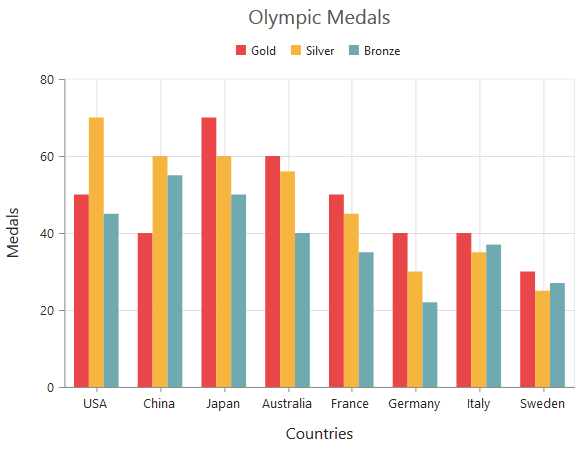### Display labels after a fixed interval

To display the labels after a fixed interval n, you can set the `interval` property of the axis range as n. The default value of the interval is 1 i.e. all the labels are displayed.

• javascript
• ``````"use strict";
var primaryXAxis={
//Displaying labels after 2 intervals
range: { interval: 2 },
};
ReactDOM.render(
<EJ.Chart id="default_chart_sample_0"
primaryXAxis= {primaryXAxis}
>

</EJ.Chart>,
document.getElementById('chart')
);``````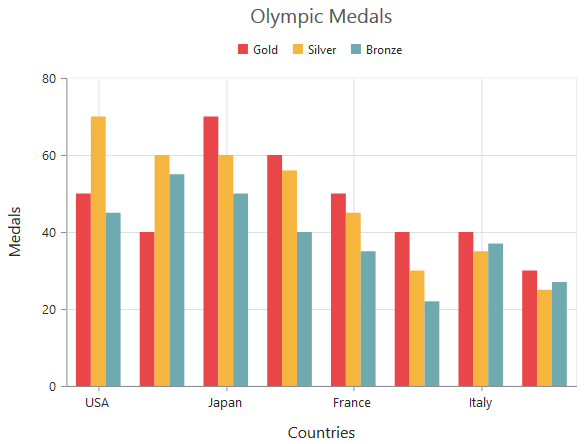### Indexed Category Axis

Category axis can also plot points based on index value of data points. Index based plotting can be enabled by setting `isIndexed` property to true in the axis.

• javascript
• ``````"use strict";
var primaryXAxis={
isIndexed: true
};
var series=[
{
points:[{ x: "Monday", y: 50 }, { x: "Tuesday", y: 40 }, { x: "Wednesday", y: 70 },
{ x: "Thursday", y: 60 }, { x: "Friday", y: 50 },
{ x: "Monday", y: 40 }, { x: "Monday", y: 30 }
]
}
];
ReactDOM.render(
<EJ.Chart id="default_chart_sample_0"
primaryXAxis= {primaryXAxis}
series = {series}
>

</EJ.Chart>,
document.getElementById('chart')
);``````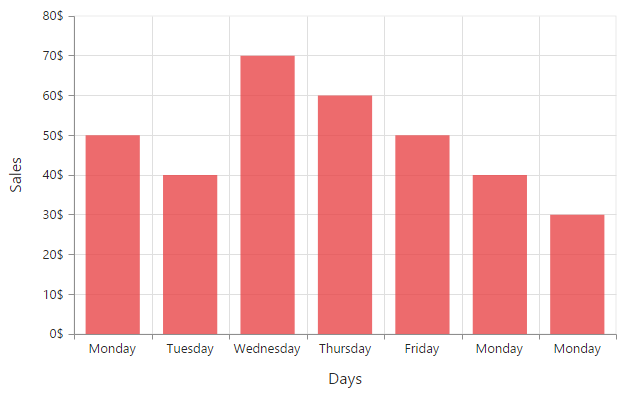While Category axis isIndexed value false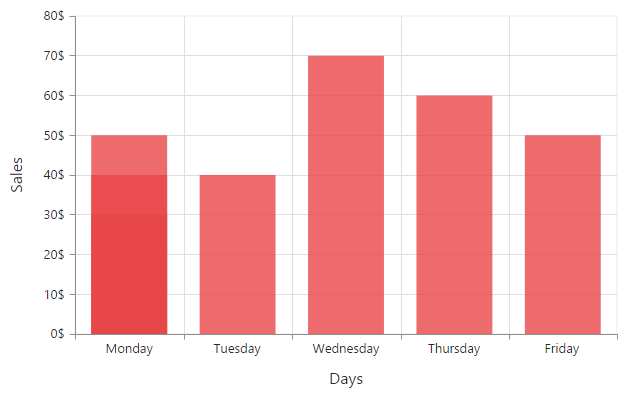## Numeric Axis

Numeric axis uses numerical scale and displays numbers as labels. To use numeric axis, you can set the `valueType` property of the axis to double.

• javascript
• ``````"use strict";
var primaryYAxis={
//Use numerical scale in primary Y axis
valueType: 'double',
};

ReactDOM.render(
<EJ.Chart id="default_chart_sample_0"
primaryYAxis= {primaryYAxis}>

</EJ.Chart>,
document.getElementById('chart')
);``````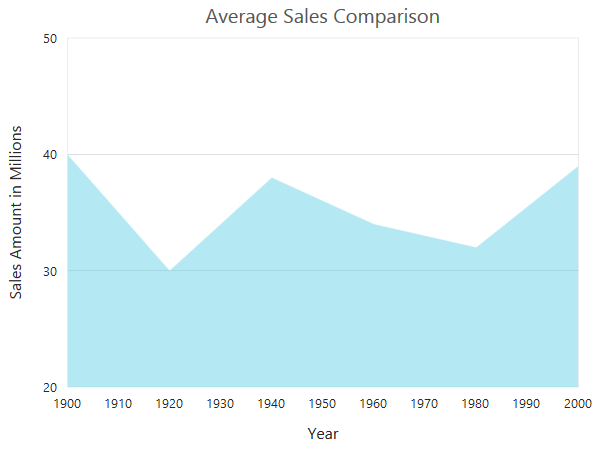### Customize numeric range

To customize the range of an axis, you can use the `range` property of the axis to set the `minimum`, `maximum` and `interval` values. Nice range is calculated automatically based on the provided data, by default.

• javascript
• ``````"use strict";
var primaryYAxis={
//Customizing Y-axis range
range: { min: 0, max: 50 },
};
ReactDOM.render(
<EJ.Chart id="default_chart_sample_0"
primaryYAxis= {primaryYAxis}
>

</EJ.Chart>,
document.getElementById('chart')
);``````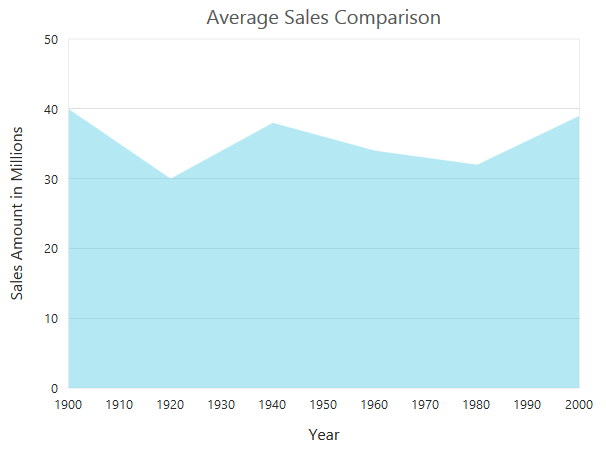#### Customizing numeric interval

Axis interval can be customized by using the `interval` property of the axis range. Nice interval is calculated based on the minimum and maximum value of the provided data, by default.

• javascript
• ``````"use strict";
var primaryYAxis={
//Customizing Y-axis interval
range: { interval: 5 },
};
ReactDOM.render(
<EJ.Chart id="default_chart_sample_0"
primaryYAxis= {primaryYAxis}
>

</EJ.Chart>,
document.getElementById('chart')
);``````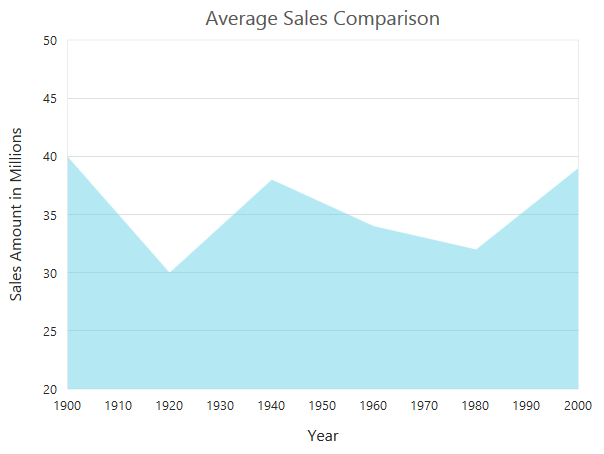### Apply padding to the range

Padding can be applied to the minimum and maximum extremes of the axis range by using the `rangePadding` property. Numeric axis supports the following types of padding

• None
• Round
• Normal

None

When the value of the `rangePadding` property is none, padding can not be applied to the axis. This is also the default value of the rangePadding.

• javascript
• ``````"use strict";
var primaryYAxis={
};
ReactDOM.render(
<EJ.Chart id="default_chart_sample_0"
primaryYAxis= {primaryYAxis}
>

</EJ.Chart>,
document.getElementById('chart')
);``````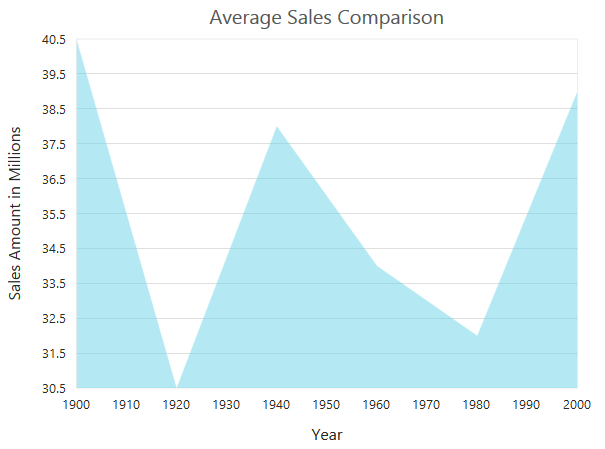#### Round

When the value of `rangePadding` property is round, the axis range is rounded to the nearest possible value divided by the interval.

• javascript
• ``````"use strict";
var primaryYAxis={
};
ReactDOM.render(
<EJ.Chart id="default_chart_sample_0"
primaryYAxis= {primaryYAxis}
>

</EJ.Chart>,
document.getElementById('chart')
);``````

Chart before rounding axis rangeChart after rounding axis range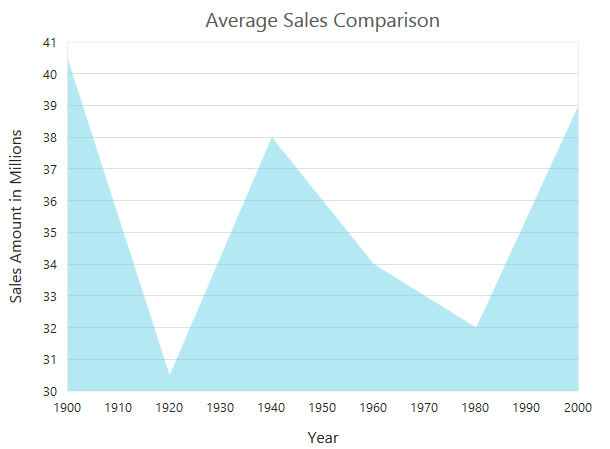When the value of the `rangePadding` property is additional, the axis range is rounded and an interval of the axis is added as padding to the minimum and maximum values of the range.

• javascript
• ``````"use strict";
var primaryYAxis={
};
ReactDOM.render(
<EJ.Chart id="default_chart_sample_0"
primaryYAxis= {primaryYAxis}
>

</EJ.Chart>,
document.getElementById('chart')
);``````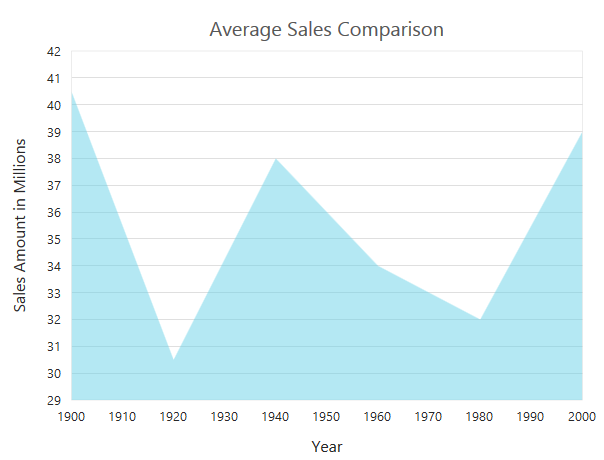Normal

When the value of the `rangePadding` property is normal, the padding is applied to the axis based on the range calculation.

• javascript
• ``````"use strict";
var primaryYAxis={
};
ReactDOM.render(
<EJ.Chart id="default_chart_sample_0"
primaryYAxis= {primaryYAxis}
>

</EJ.Chart>,
document.getElementById('chart')
);``````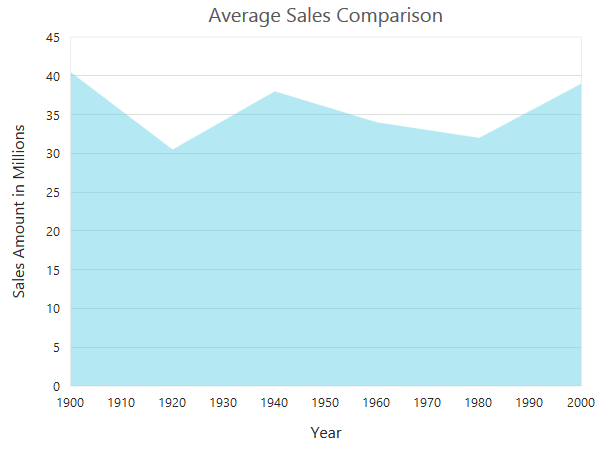####Customizing the starting range of the axis

By default the Y axis will be always calculated from the value 0 for column, bar, stacking column and stacking bar series types. You can modify this behavior by setting false to the property `startFromZero` in the axis. On setting this the axis minimum value will be calculated based on the value for the data points.

• javascript
• ``````"use strict";
//Initializing Primary Y Axis
var primaryYAxis={
startFromZero: false
};

ReactDOM.render(
<EJ.Chart id="default_chart_sample_0"
primaryYAxis= {primaryYAxis}
>

</EJ.Chart>,
document.getElementById('chart')
);``````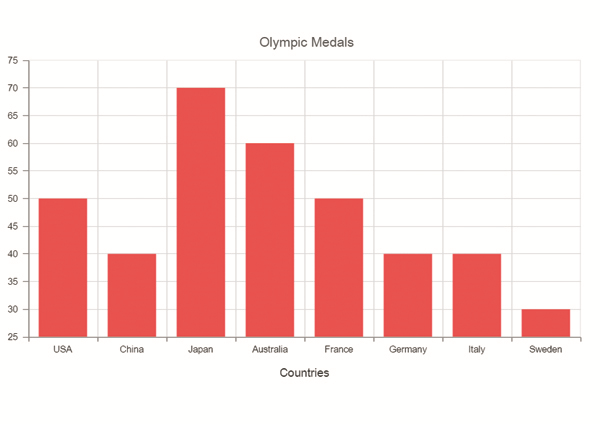## DateTime Axis

Date time axis uses date time scale and displays the date time values as axis labels in the specified format. To use date time axis, set the `valueType` property of the axis to datetime.

• javascript
• ``````"use strict";
var primaryXAxis={
//Use date time scale in primary X axis
valueType: 'datetime',
};
ReactDOM.render(
<EJ.Chart id="default_chart_sample_0"
primaryXAxis= {primaryXAxis}
>

</EJ.Chart>,
document.getElementById('chart')
);``````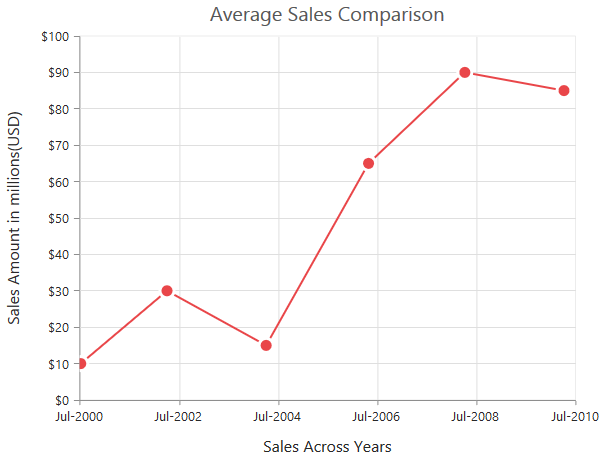Click here to view our online demo sample for date time axis.

### Customizing date time range

Axis range can be customized by using the `range` property to set the `minimum`, `maximum` and `interval` values. Nice range is calculated automatically based on the provided data, by default.

• javascript
• ``````"use strict";
var primaryXAxis={
//Customizing X-axis date time range
range: {
min: new Date(2000, 6, 1),
max: new Date(2010, 6, 1)
},
};

ReactDOM.render(
<EJ.Chart id="default_chart_sample_0"
primaryXAxis= {primaryXAxis}
>

</EJ.Chart>,
document.getElementById('chart')
);``````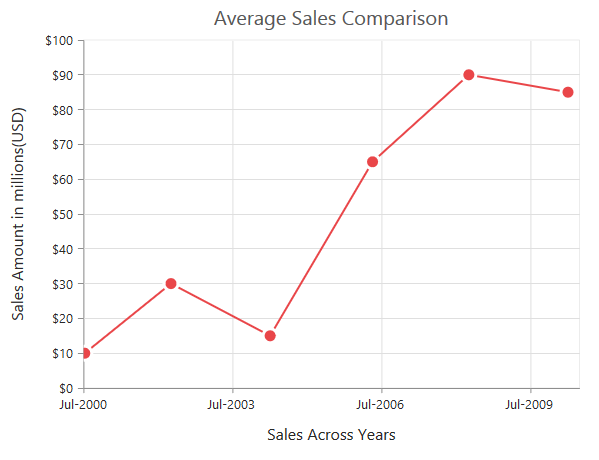### Date time intervals

Date time intervals can be customized by using the `interval` and `intervalType` properties of the axis. For example, when you set `interval` as 2 and `intervalType` as years, it considers the 2 years as interval.

Essential Chart supports the following types of interval for date time axis.

• Days
• Hours
• Milliseconds
• Minutes
• Months
• Seconds
• Years
• javascript
• ``````"use strict";
var primaryXAxis={
//Customizing X-axis date time range
range: {
interval: 2,
},

intervalType: 'years',

};
ReactDOM.render(
<EJ.Chart id="default_chart_sample_0"
primaryXAxis= {primaryXAxis}
>

</EJ.Chart>,
document.getElementById('chart')
);``````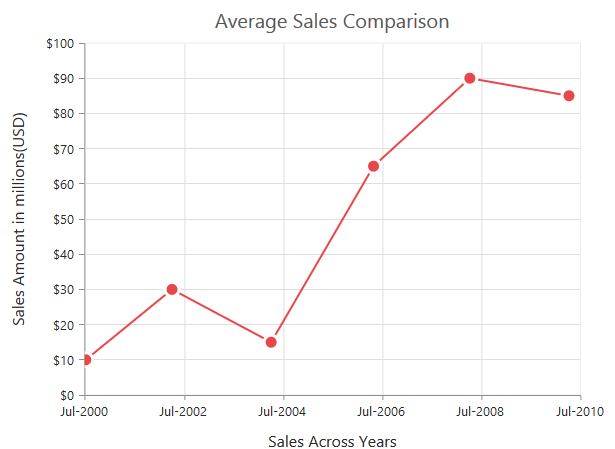### Apply padding to the range

Padding can be applied to the minimum and maximum extremes of the range by using the `rangePadding` property. Date time axis supports the following types of padding

• None
• Round

None

When the value of the `rangePadding` property is none, padding is applied to the axis. This is also the default value of the rangePadding.

• javascript
• ``````"use strict";
var primaryXAxis={

//  ...

};
ReactDOM.render(
<EJ.Chart id="default_chart_sample_0"
primaryXAxis= {primaryXAxis}
>

</EJ.Chart>,
document.getElementById('chart')
);``````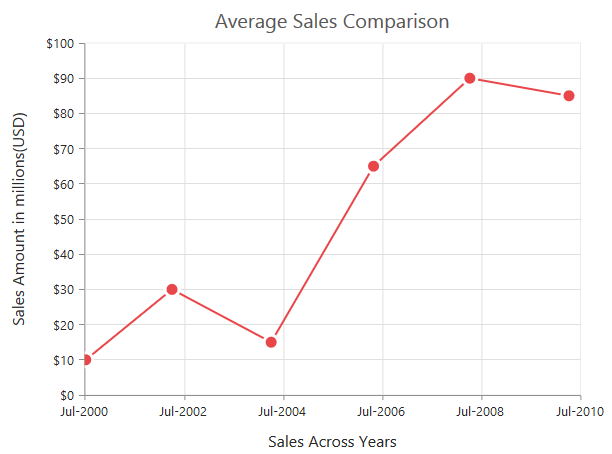Round

When the value of the `rangePadding` property is round, the axis range is rounded to the nearest possible date time value.

• javascript
• ``````"use strict";
var primaryXAxis={

//  ...

};
ReactDOM.render(
<EJ.Chart id="default_chart_sample_0"
primaryXAxis= {primaryXAxis}
>

</EJ.Chart>,
document.getElementById('chart')
);``````

Chart before rounding axis range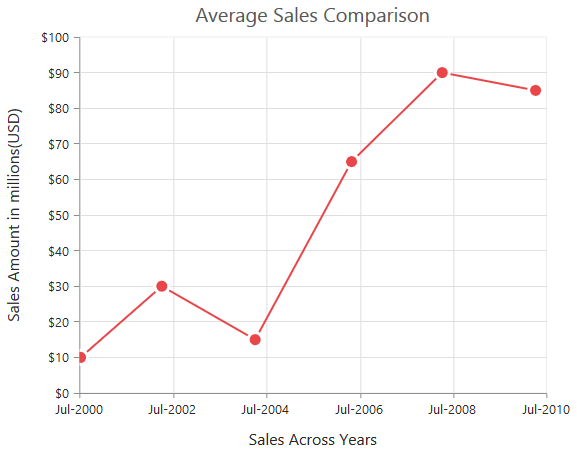Chart after rounding axis range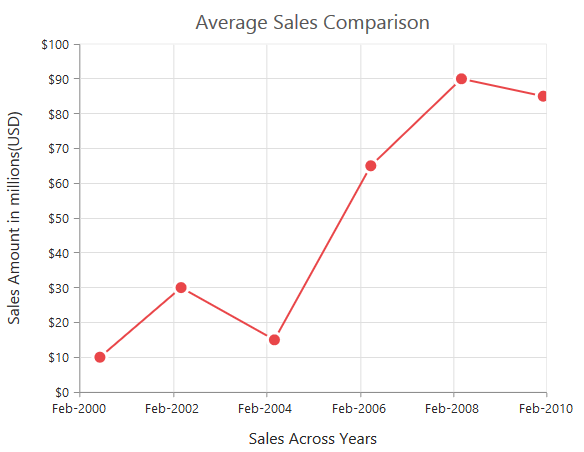When the value of the `rangePadding` property is additional, the range is rounded and date time interval of the axis are added as padding to the minimum and maximum extremes of the range.

• javascript
• ``````"use strict";
var primaryXAxis={

//  ...

};
ReactDOM.render(
<EJ.Chart id="default_chart_sample_0"
primaryXAxis= {primaryXAxis}
>

</EJ.Chart>,
document.getElementById('chart')
);``````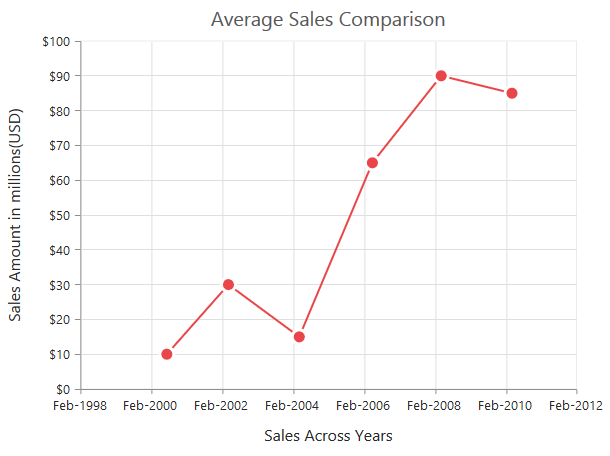## DateTime Category Axis

DateTime category axis takes date time value as input but behaves like category axis. This is used to display the date time values with nonlinear intervals (used to depict the business days by skipping holidays). To use date time axis, set the [`valueType`] (../api/ejchart#members:primaryxaxis-valuetype) property of the axis to datetimeCategory.

• javascript
• ``````"use strict";
var primaryXAxis={

valueType: 'datetimeCategory',
// ...

};
ReactDOM.render(
<EJ.Chart id="default_chart_sample_0"
primaryXAxis= {primaryXAxis}
>

</EJ.Chart>,
document.getElementById('chart')
);``````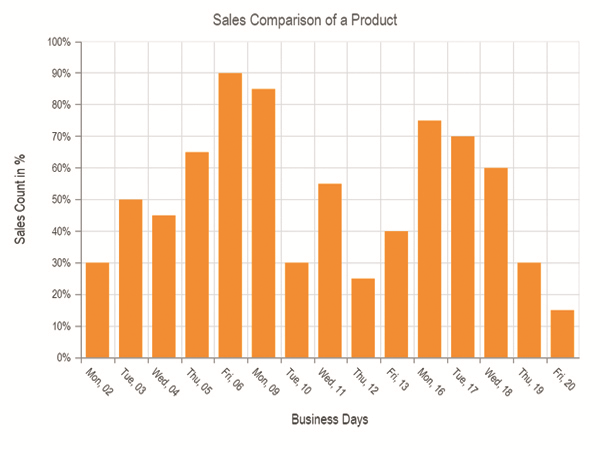Click here to view our online demo sample for date time axis.

### Customizing DateTime Category range

Axis range can be customized by using the `range` property to set the `minimum`, `maximum` and `interval` values. Datetime category axis takes numeric input for minimum and maximum property.

• javascript
• ``````"use strict";
var primaryXAxis={

range: {  //Customizing X-axis date time category range
min: 0,
max: 4
},

//  ...

};
ReactDOM.render(
<EJ.Chart id="default_chart_sample_0"
primaryXAxis= {primaryXAxis}
>

</EJ.Chart>,
document.getElementById('chart')
);``````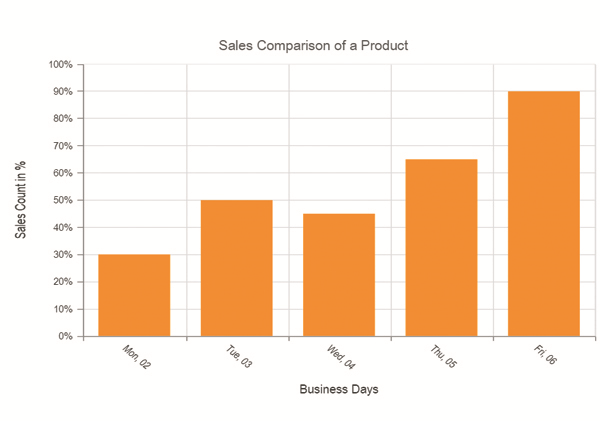### DateTime Category intervals

Date time category intervals can be customized by using the `interval` and `intervalType` properties of the axis. For example, when you set the intervalType as months, it displays only the first label of all the months from the data.

Essential Chart supports the following types of interval for date time category axis.

• Days
• Hours
• Milliseconds
• Minutes
• Months
• Seconds
• Years
• Auto
• javascript
• ``````"use strict";
var primaryXAxis={

intervalType: "months"
//  ...

};
ReactDOM.render(
<EJ.Chart id="default_chart_sample_0"
primaryXAxis= {primaryXAxis}
>

</EJ.Chart>,
document.getElementById('chart')
);``````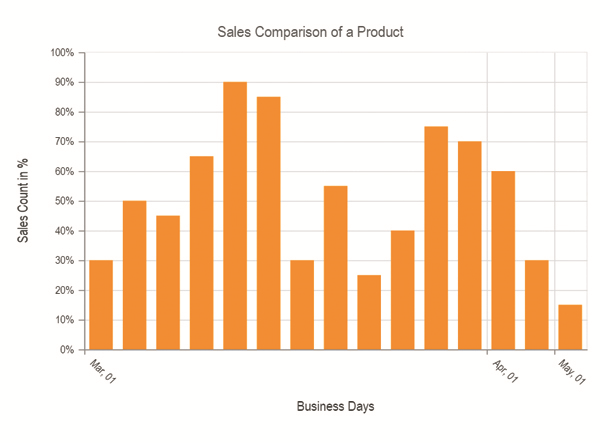## Logarithmic Axis

Logarithmic axis uses logarithmic scale and it is very useful in visualizing when the data has values with both lower order of magnitude (eg: 10-6) and higher order of magnitude (eg: 106). To use logarithmic axis, set the `valueType` property of the axis to logarithmic.

• javascript
• ``````"use strict";
var primaryXAxis={

//Use logarithmic scale in primary X axis
valueType: 'logarithmic',

//  ...

};
ReactDOM.render(
<EJ.Chart id="default_chart_sample_0"
primaryXAxis= {primaryXAxis}
>

</EJ.Chart>,
document.getElementById('chart')
);``````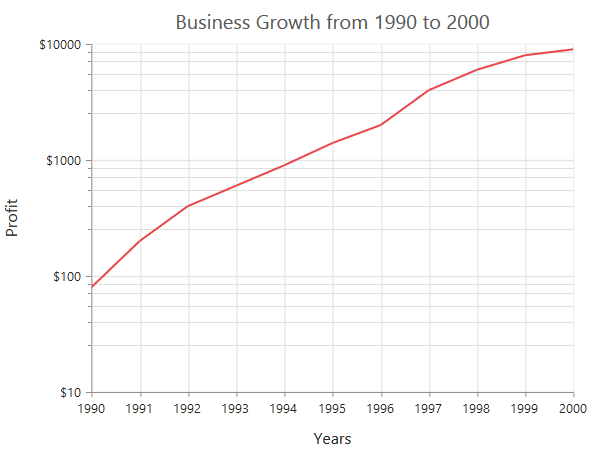### Customize Logarithmic range

Logarithmic range can be customized by using the `range` property of the axis to change the `minimum`, `maximum` and `interval` values. Nice range is calculated automatically based on the provided data, by default.

• javascript
• ``````"use strict";
var primaryXAxis={

//Customizing logarithmic range
range: { min: 1, max: 5 },

//  ...

};
ReactDOM.render(
<EJ.Chart id="default_chart_sample_0"
primaryXAxis= {primaryXAxis}
>

</EJ.Chart>,
document.getElementById('chart')
);``````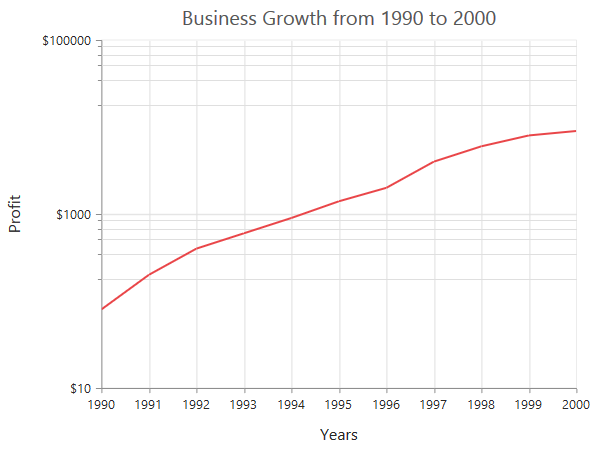### Logarithmic base

Logarithmic base can be customized by using the `logBase` property of the axis. The default value of the `logBase` is 10.

• javascript
• ``````"use strict";
var primaryXAxis={

//Customizing logarithmic base
logBase: 2,
//  ...

};
ReactDOM.render(
<EJ.Chart id="default_chart_sample_0"
primaryXAxis= {primaryXAxis}
>

</EJ.Chart>,
document.getElementById('chart')
);``````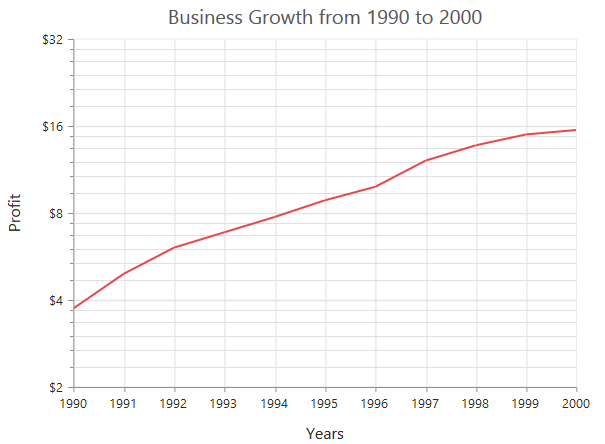### Logarithmic interval

Logarithmic axis interval can be customized by using the `interval` property of the axis. When the logarithmic base is 10 and logarithmic interval is 2, then the axis labels are placed at an interval of 102. The default value of the interval is 1.

• javascript
• ``````"use strict";
var primaryXAxis={

//Customizing logarithmic interval
range: { interval: 2 },
//  ...

};
ReactDOM.render(
<EJ.Chart id="default_chart_sample_0"
primaryXAxis= {primaryXAxis}
>

</EJ.Chart>,
document.getElementById('chart')
);``````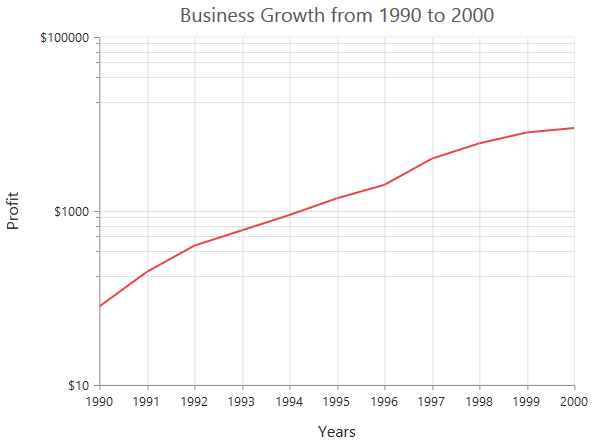## Label Format

### Format numeric labels

Numeric labels can be formatted by using the `labelFormat` property. Numeric values can be formatted with n (number with decimal points), c (currency) and p (percentage) commands.

• javascript
• ``````"use strict";
var primaryXAxis={
//Applying currency format to axis labels
labelFormat: 'c',
//  ...
};
ReactDOM.render(
<EJ.Chart id="default_chart_sample_0"
primaryXAxis= {primaryXAxis}
>

</EJ.Chart>,
document.getElementById('chart')
);``````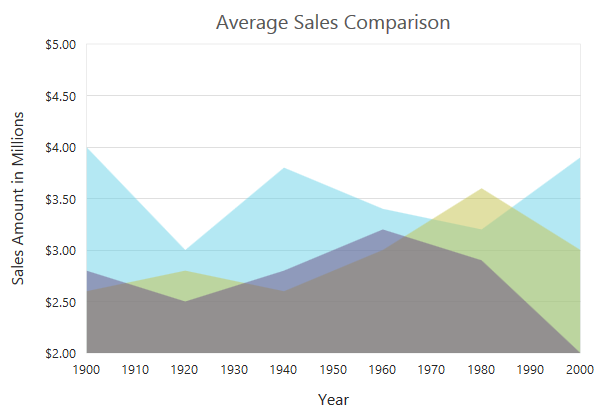The following table describes the result of applying some commonly used label formats on numeric values.

 Label Value Label Format property value Result Description 1000 n1 1000.0 The Number is rounded to 1 decimal place 1000 n2 1000.00 The Number is rounded to 2 decimal place 1000 n3 1000.000 The Number is rounded to 3 decimal place 0.01 p1 1.0% The Number is converted to percentage with 1 decimal place 0.01 p2 1.00% The Number is converted to percentage with 2 decimal place 0.01 p3 1.000% The Number is converted to percentage with 3 decimal place 1000 c1 \$1,000.0 The Currency symbol is appended to number and number is rounded to 1 decimal place 1000 c2 \$1,000.00 The Currency symbol is appended to number and number is rounded to 2 decimal place

### Format date time values

Date time labels can be formatted by using the `labelFormat` property of the axis.

• javascript
• ``````"use strict";
var primaryXAxis={
//Formatting date time labels in date/Month name/Year format
labelFormat: 'dd/MMMM/yyyy',
//  …
};
ReactDOM.render(
<EJ.Chart id="default_chart_sample_0"
primaryXAxis= {primaryXAxis}
>

</EJ.Chart>,
document.getElementById('chart')
);``````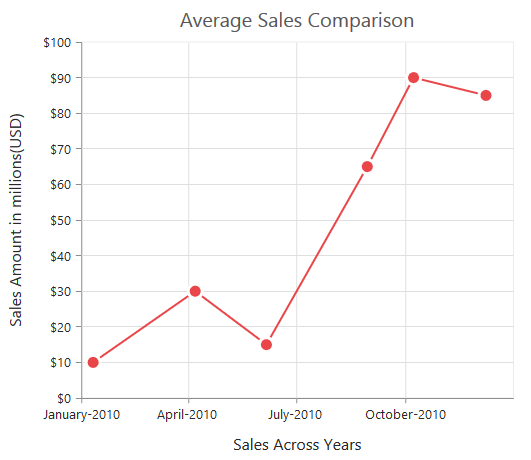The following table describes the result of applying some common date time formats to the labelFormat property

 Label Value Label Format Property Value Result Description new Date(2000, 03, 10) dddd Monday The Date is displayed in day format new Date(2000, 03, 10) MM/dd/yyyy 04/10/2000 The Date is displayed in month/date/year format new Date(2000, 03, 10) n3 1000.000 The Number is rounded to 3 decimal place new Date(2000, 03, 10) MMM Apr The Shorthand month for the date is displayed new Date(2000, 03, 10) t 12:00 AM Time of the date value is displayed as label new Date(2000, 03, 10) hh:mm:ss 12:00:00 The Label is displayed in hours:minutes:seconds format

### Custom label format

Prefix and suffix can be added to the category labels by using the `labelFormat` property. You can use the {value} as placeholder text in your custom text, it is replaced with the corresponding axis label at the runtime.

• javascript
• ``````"use strict";
var primaryXAxis={
//...
//Adding prefix and suffix to axis labels
labelFormat: '\${value} K',
};
ReactDOM.render(
<EJ.Chart id="default_chart_sample_0"
primaryXAxis= {primaryXAxis}
>

</EJ.Chart>,
document.getElementById('chart')
);``````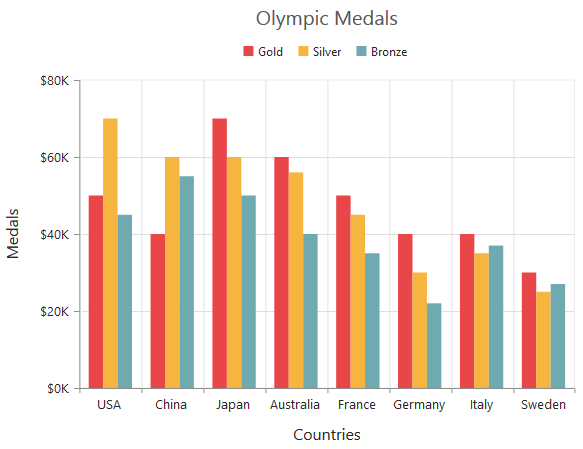## Common axis features

Customization of features such as axis crossing, title, labels, grid lines and tick lines are common to all the axis. Each of these features are explained in this section.

### Axis Crossing

Axis can be positioned anywhere in chart area using the `crossesAt` property of axis. This property specifies where the horizontal axis should intersect or cross the vertical axis and vice versa. Default value of `crossesAt` property is null.

• javascript
• ``````"use strict";
var primaryXAxis={
//Crosses primary Y axis at 0
crossesAt: 0,

//...
};
ReactDOM.render(
<EJ.Chart id="default_chart_sample_0"
primaryXAxis= {primaryXAxis}
>

</EJ.Chart>,
document.getElementById('chart')
);``````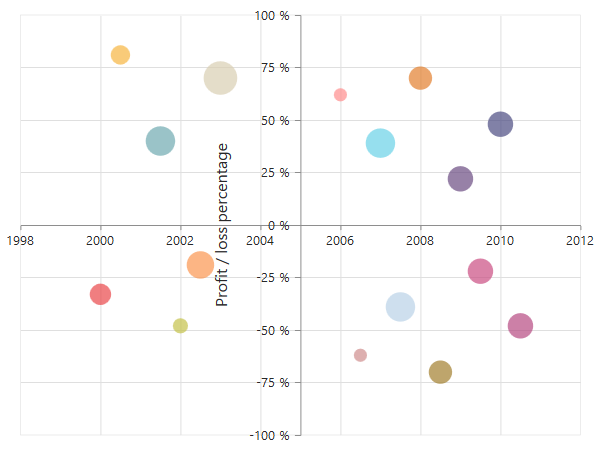#### Crossing a specific Axis

The `crossesInAxis` property takes axis name as input and determines the axis used for crossing. By default all the horizontal axes crosses in primary Y axis and all the vertical axes crosses in primary X axis.

• javascript
• ``````"use strict";
var primaryXAxis={
//Crosses vertical axis at -0.2
crossesAt: -0.2,

//Crosses in secondary Y axis
crossesInAxis: 'secondaryYAxis',

//...
};
var axes=[{
orientation: 'vertical',
name: 'secondaryYAxis',

//...
}];
ReactDOM.render(
<EJ.Chart id="default_chart_sample_0"
primaryXAxis= {primaryXAxis}
axes={axes}
>

</EJ.Chart>,
document.getElementById('chart')
);``````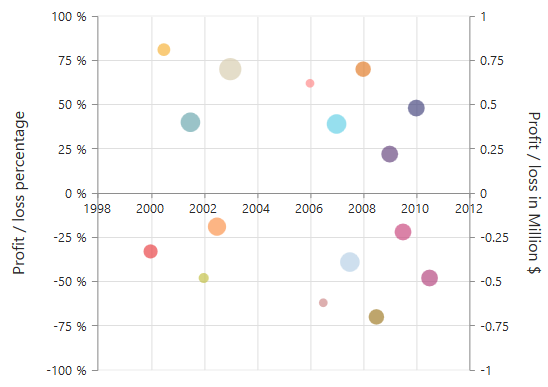Axis will be placed in the opposite side if value of `crossesAt` property is greater than the maximum value of crossing axis (axis name provided through `crossesInAxis` property or primary Y axis for horizontal axis).

• javascript
• ``````"use strict";
var primaryXAxis={
//Crosses primary Y axis at 200
crossesAt: 200,

//...
};
ReactDOM.render(
<EJ.Chart id="default_chart_sample_0"
primaryXAxis= {primaryXAxis}
>

</EJ.Chart>,
document.getElementById('chart')
);``````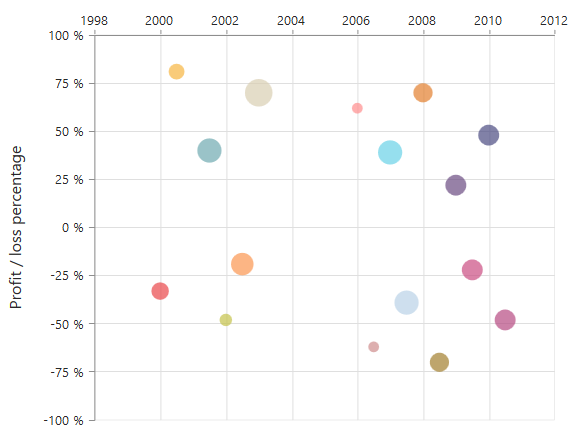#### Crossing in DateTime Axis

For crossing in a date time horizontal axis, date object should be provided as value for `crossesAt` property of vertical axes.

• javascript
• ``````"use strict";
var primaryYAxis={
//Crosses horizontal axis at 5/29/2010
crossesAt: new Date(2010, 4, 29),

//...
};
ReactDOM.render(
<EJ.Chart id="default_chart_sample_0"
primaryYAxis= {primaryYAxis}
>

</EJ.Chart>,
document.getElementById('chart')
);``````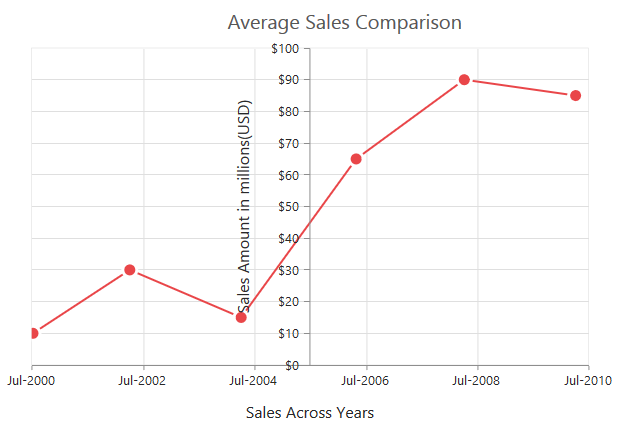#### Crossing in Category Axis

For crossing in a category type horizontal axis, either a string object or a number corresponding to the index of category value can be used for `crossesAt` property of vertical axes.

WARNING

String value provided for `crossesAt` property is case-sensitive.

• javascript
• ``````"use strict";
var primaryYAxis={
//Crosses horizontal axis at category value ‘Third’
crossesAt: 'Tuesday',

//...
};
ReactDOM.render(
<EJ.Chart id="default_chart_sample_0"
primaryYAxis= {primaryYAxis}
>

</EJ.Chart>,
document.getElementById('chart')
);``````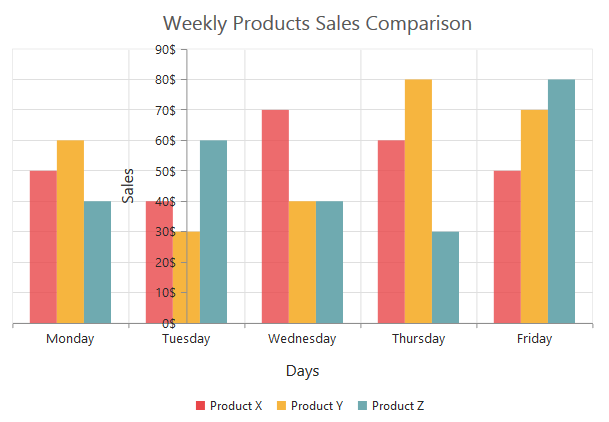#### Positioning the axis elements while crossing

The `showNextToAxisLine` property is used for controlling the axis elements movement along with the axis line while axis crossing is performed. When the showNextToAxisLine is set as false only the axis line and the tick lines are placed at the crossing Value and the axis elements will be placed outside the chart area. The default value of `showNextToAxisLine` is true.

• javascript
• ``````var primaryXAxis = {
//Crosses primary Y axis at 0
crossesAt: 0,
showNextToAxisLine:false,
//...
}

ReactDOM.render(
<EJ.Chart id="default_chart_sample_0"
width="100%"
primaryXAxis={primaryXAxis}>
</EJ.Chart>,
document.getElementById('chart')
);``````

The axis is placed at the crossing value without the axis elements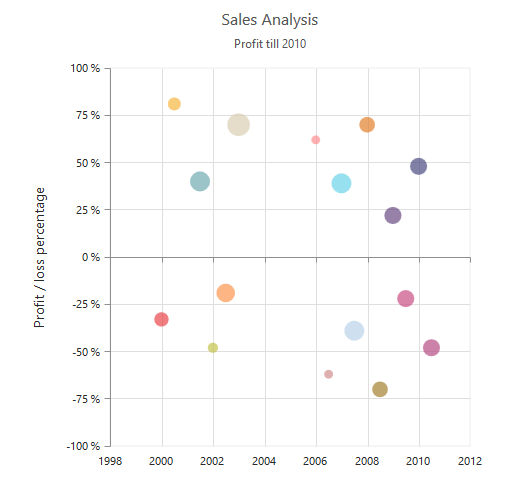### Axis Visibility

Axis visibility can be controlled by using the `visible` property of the axis. The default value of the `visible` property is true.

• javascript
• ``````"use strict";
var primaryYAxis={
//Disabling visibility of Y-axis
visible: false

//  ...
};
ReactDOM.render(
<EJ.Chart id="default_chart_sample_0"
primaryYAxis= {primaryYAxis}
>

</EJ.Chart>,
document.getElementById('chart')
);``````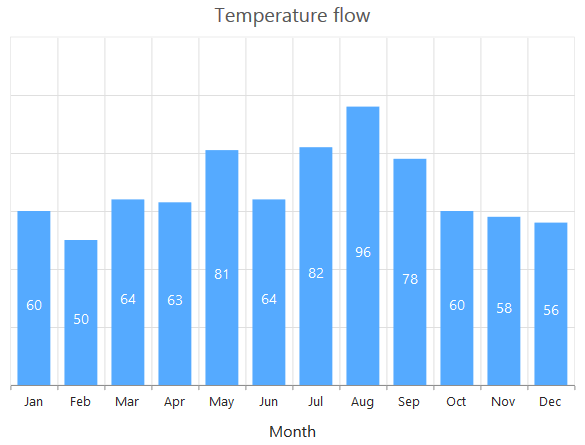### Axis title

The `title` property in the axis provides options to customize the text and font of the axis title. Axis does not display the title, by default. Title text can also be trimmed based on the title text length or specified length.

• javascript
• ``````"use strict";
var primaryXAxis = {
//Customizing axis title
title : {
text : 'Month',
font : {
fontFamily : 'Segoe UI',
size : '16px',
fontWeight : 'bold' ,
color : 'grey',
},
enableTrim : true,
maximumTitleWidth : 80
}
//  ...
}
ReactDOM.render(
<EJ.Chart id="default_chart_sample_0"
primaryXAxis= {primaryXAxis}
>

</EJ.Chart>,
document.getElementById('chart')
);``````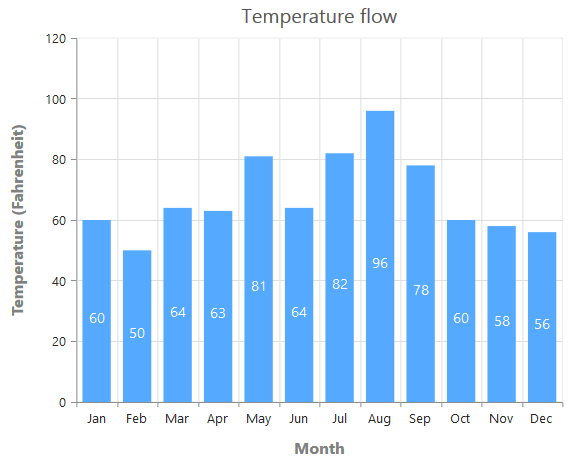You can modify the position of the axis title either inside or outside the chart area using the property [`position`]. By default, it will be placed outside the chart area. In addition, you can also change the alignment of the title to near, far and center by [`alignment`] property, using [`offset`] property you can change the position with respect to pixels.

• javascript
• ``````"use strict";
var primaryXAxis = {
//Customizing axis title
title : {
text : 'Month',
position:'inside',
alignment:'near',
offset: 10
}
//  ...
}
ReactDOM.render(
<EJ.Chart id="default_chart_sample_0"
primaryXAxis= {primaryXAxis}
>

</EJ.Chart>,
document.getElementById('chart')
);``````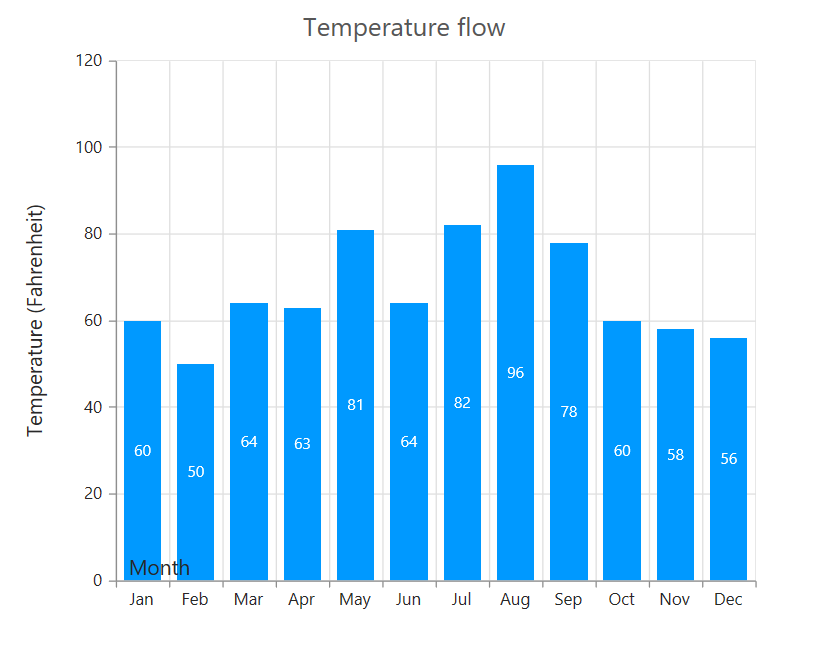### Label customization

The `font` property of the axis provides options to customize the `font-family`, `color`, `opacity`, `size` and `font-weight` of the axis labels.

• javascript
• ``````"use strict";
var primaryXAxis = {
//Customizing label appearance
font : {
fontFamily : 'Segoe UI',
size : '14px',
fontWeight : 'bold' ,
color : 'blue',
},
//  ...
}
ReactDOM.render(
<EJ.Chart id="default_chart_sample_0"
primaryXAxis= {primaryXAxis}
>

</EJ.Chart>,
document.getElementById('chart')
);``````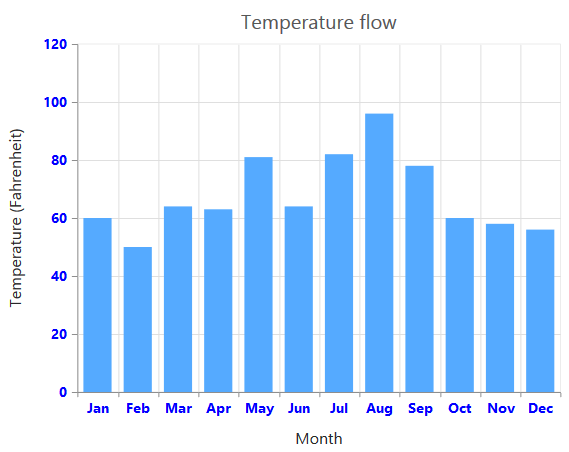### Label and tick positioning

Axis labels and ticks can be positioned inside or outside the chart area by using the `labelPosition` and `tickPosition` properties. The labels and ticks are positioned outside the chart area, by default.

• javascript
• ``````"use strict";
var primaryXAxis = {
//Customizing label and tick positions
labelPosition : 'inside',
tickLinesPosition : 'inside',

//  ...
}
ReactDOM.render(
<EJ.Chart id="default_chart_sample_0"
primaryXAxis= {primaryXAxis}
>

</EJ.Chart>,
document.getElementById('chart')
);``````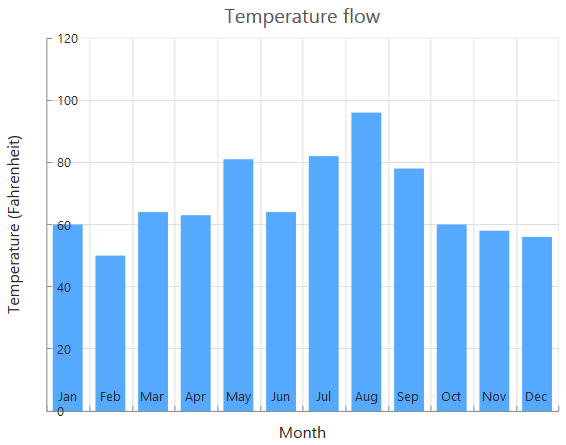### Edge labels placement

Labels with long text at the edges of an axis may appear partially outside the chart. The `edgeLabelPlacement` property can be used to avoid the partial appearance of the labels at the corners.

• javascript
• ``````"use strict";
var primaryXAxis = {
//Customizing edge label placement
edgeLabelPlacement : 'shift',
//  ...
}
ReactDOM.render(
<EJ.Chart id="default_chart_sample_0"
primaryXAxis= {primaryXAxis}
>

</EJ.Chart>,
document.getElementById('chart')
);``````

Chart before setting edge label placement to X-axis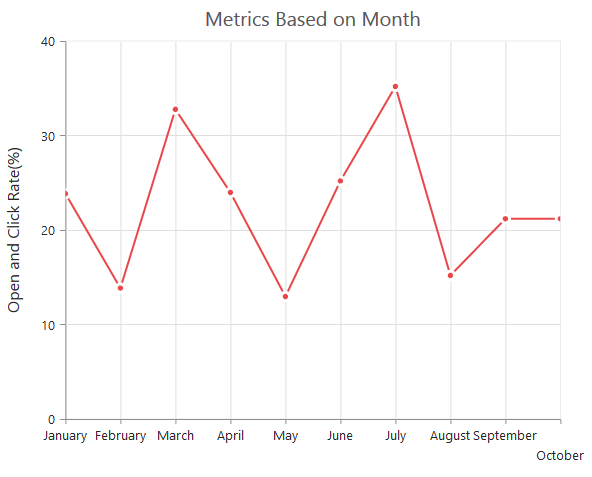Chart after setting edge label placement to X-axis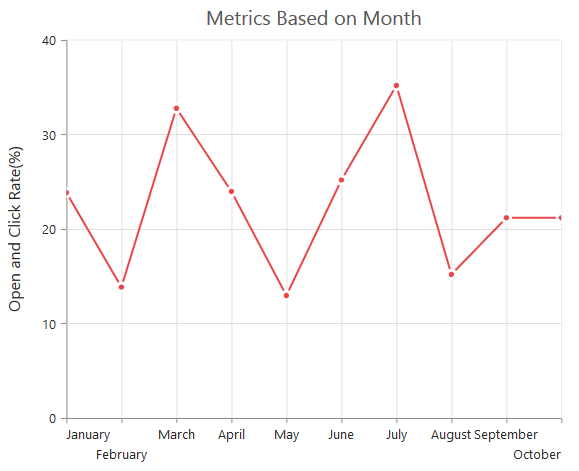### Grid lines customization

The `majorGridLines` and `minorGridLines` properties in the axis are used to customize the major grid lines and minor grid lines of an axis. They provide options to change the width, color, visibility and opacity of the grid lines. The minor grid lines are not visible, by default.

• javascript
• ``````"use strict";
var primaryXAxis = {
//Customizing grid lines
majorGridLines : {
color : 'blue',
visible : true,
width : 1
},
minorTicksPerInterval: 0,
minorGridLines : {
color : 'red',
visible : false,
width : 1
}
}
//  ...

ReactDOM.render(
<EJ.Chart id="default_chart_sample_0"
primaryXAxis= {primaryXAxis}
>

</EJ.Chart>,
document.getElementById('chart')
);``````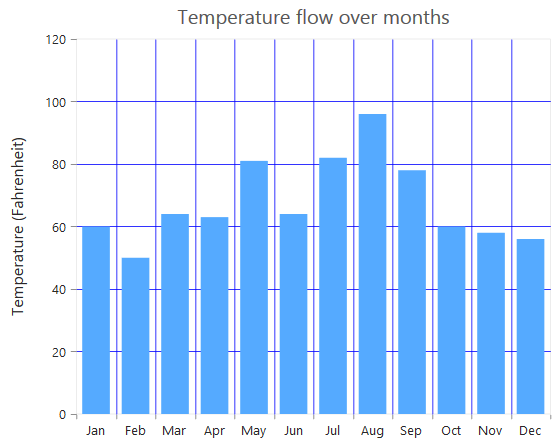### Tick lines customization

The `majorTickLines` and `minorTickLines` properties in the axis are used to customize the major tick lines of an axis and minor tick lines of an axis. They provide options to change the width, size, color and visibility of the grid lines. The minor tick lines are not visible, by default.

• javascript
• ``````"use strict";
var primaryXAxis = {
//Customizing tick lines
majorTickLines : {
color : 'blue',
visible : true,
width : 1 ,
size : 5,
},
minorTicksPerInterval: 0,
minorTickLines : {
color : 'red',
visible : false,
width : 1 ,
size : 5
}
//  ...
}

ReactDOM.render(
<EJ.Chart id="default_chart_sample_0"
primaryXAxis= {primaryXAxis}
>

</EJ.Chart>,
document.getElementById('chart')
);``````### Inversing axis

Axis can be inversed by using the `isInversed` property of the axis. The default value of the `isInversed` property is false.

• javascript
• ``````"use strict";
var primaryXAxis = {
//Inversing the X-axis
isInversed: false

//  ...
}

ReactDOM.render(
<EJ.Chart id="default_chart_sample_0"
primaryXAxis= {primaryXAxis}
>

</EJ.Chart>,
document.getElementById('chart')
);``````

Chart before inversing the axes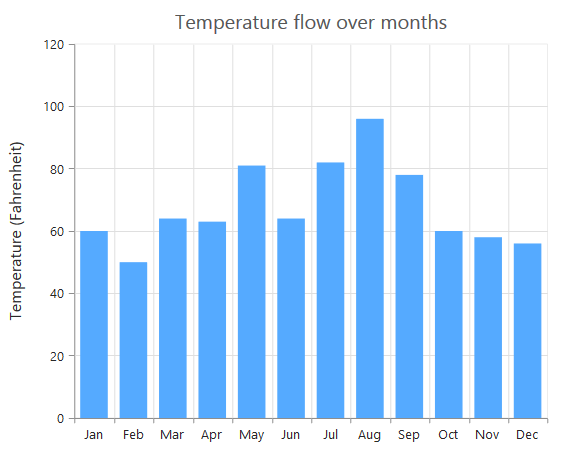Chart after inversing the axes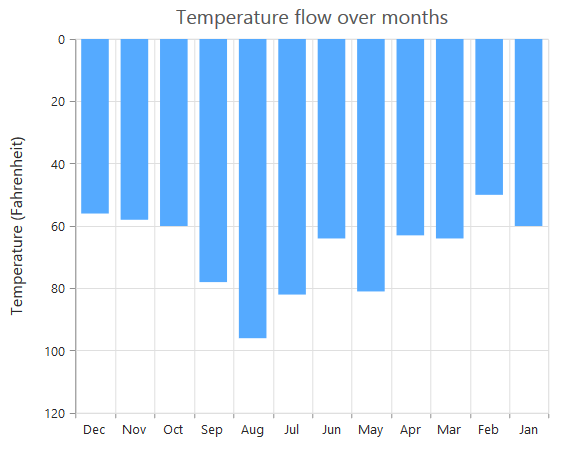### Place axes at the opposite side

The `opposedPosition` property of axis can be used to place the axis at the opposite side of its default position. The default value of the `opposedPosition` property is false.

• javascript
• ``````"use strict";
var primaryXAxis = {
//Placing axis at the opposite side of its normal position
opposedPosition: true

//  ...
}

ReactDOM.render(
<EJ.Chart id="default_chart_sample_0"
primaryXAxis= {primaryXAxis}
>

</EJ.Chart>,
document.getElementById('chart')
);``````

Chart with X and Y axes at normal position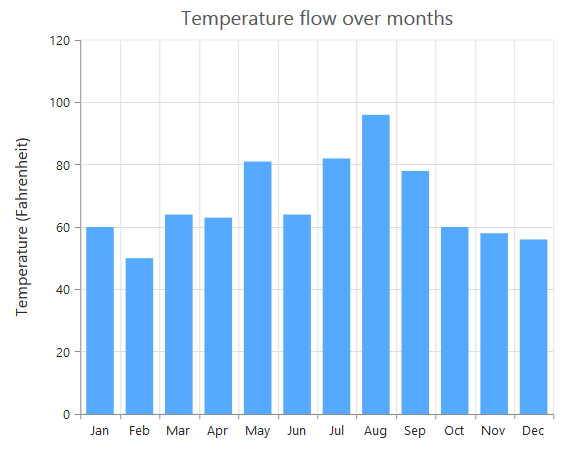Chart with Y-axis at opposed position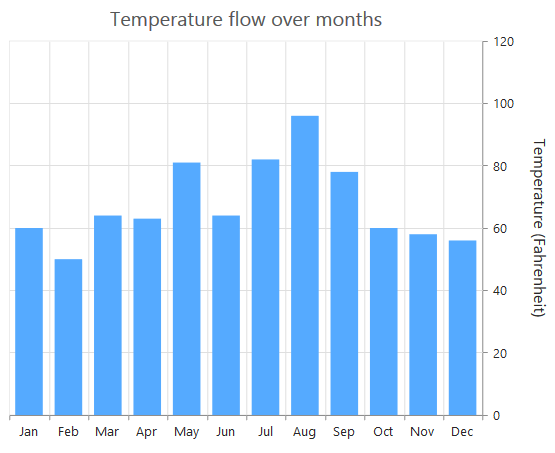### Maximum number of labels per 100 pixels

A maximum of 3 labels are displayed for each 100 pixels in the axis, by default. The maximum number of labels that is present within the 100 pixels length can be customized by using the `maximumLabels` property of the axis. This property is applicable only for an automatic range calculation and it does not work when you set the value for `interval` property in the axis range.

• javascript
• ``````"use strict";
var primaryXAxis = {
//Maximum number of labels per 100 pixels
maximumLabels : 1

//  ...
}

ReactDOM.render(
<EJ.Chart id="default_chart_sample_0"
primaryXAxis= {primaryXAxis}
>

</EJ.Chart>,
document.getElementById('chart')
);``````

Chart before setting maximum labels per 100 pixels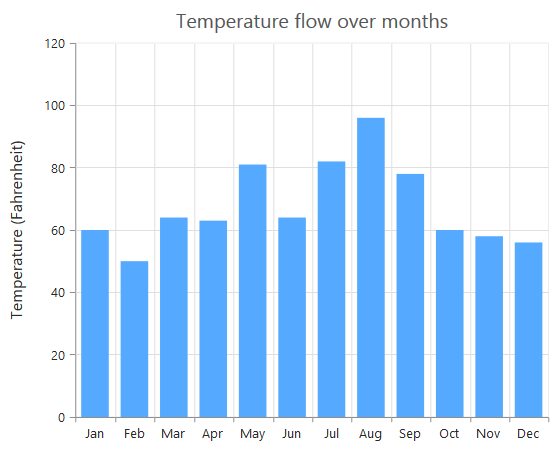Chart after setting maximum labels one per 100 pixels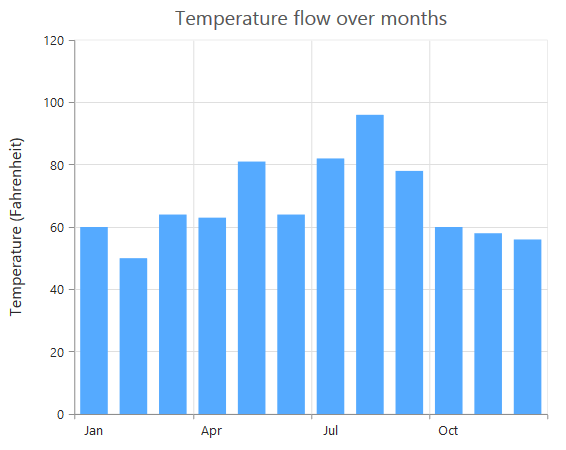## Multiple Axis

Multiple axes can be used in the Chart and chart area can be split into multiple panes to draw multiple series with multiple axes.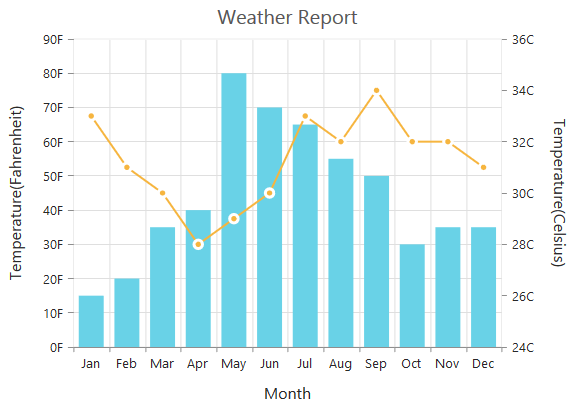An additional horizontal or vertical axis can be added to the chart by adding an axis instance to the axes collection and then you can associate it to a series by specifying the name of the axis to the `xAxisName` or `yAxisName` property of the series.

• javascript
• ``````"use strict";
var axes = [{
name : 'SecondaryX',
//  ...
},{
name : 'SecondaryY',
//  ...
}]
var series = [{
//  Binding secondary X-axis with a series
xAxisName : 'SecondaryX',

//  Binding secondary Y-axis with a series
yAxisName : 'SecondaryY',
//  …
},
{
//  ...
}]
ReactDOM.render(
<EJ.Chart id="default_chart_sample_0"
axes={axes}
sereis={series}
>

</EJ.Chart>,
document.getElementById('chart')
);``````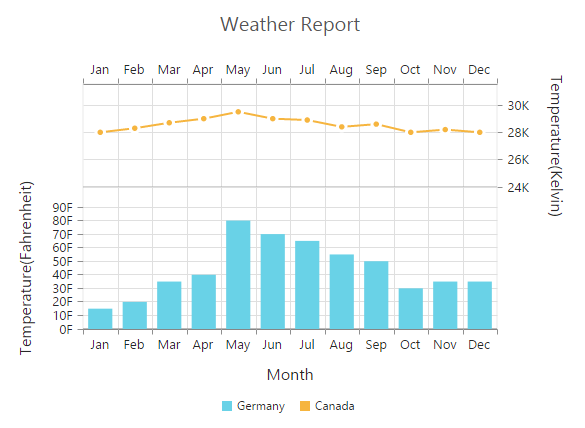## Smart Axis Labels

When the Axis labels overlap with each other based on the chart dimensions and label size, you can use the `labelIntersectAction` property of the axis to avoid overlapping. The default value of the `labelIntersectAction` is none. The other available values of the Label Intersect Actions are rotate45, rotate90, trim, multipleRows, wrap, wrapByWord and hide.

• javascript
• ``````"use strict";

var primaryXAxis = {
// Avoid overlapping of x-axis labels
labelIntersectAction : 'multipleRows',
//  ...
}

ReactDOM.render(
<EJ.Chart id="default_chart_sample_0"
primaryXAxis={primaryXAxis}
>

</EJ.Chart>,
document.getElementById('chart')
);``````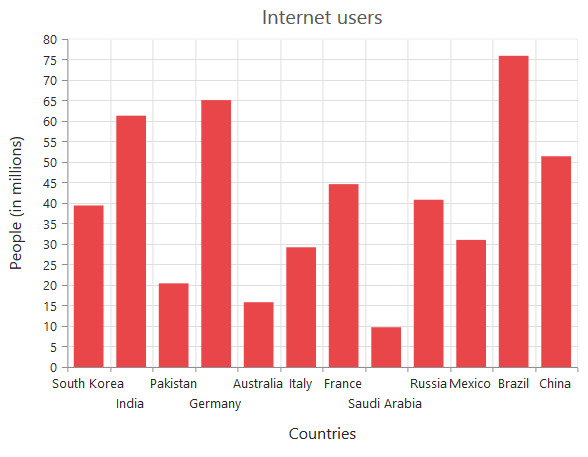Click here to view our online demo sample for smart axis labels.

The following screenshot displays the result, when the `labelIntersectAction` property is set as rotate45.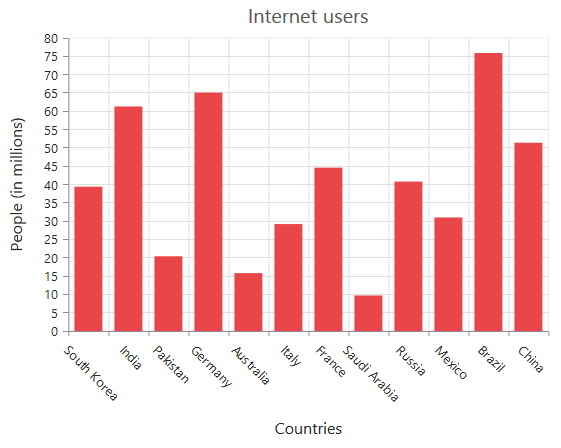The following screenshot displays the result, when the `labelIntersectAction` property is set as rotate90.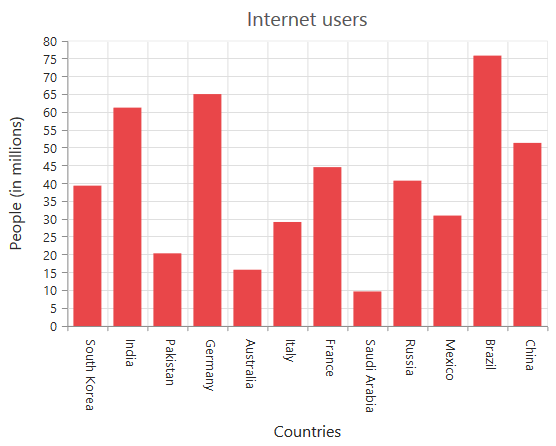The following screenshot displays the result, when the `labelIntersectAction` property is set as wrap.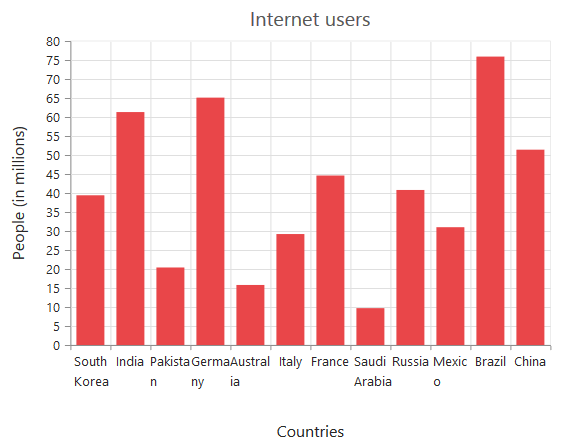The following screenshot displays the result, when of setting the trim as value to the `labelIntersectAction` property.The following screenshot displays the result, when the `labelIntersectAction` property is set as hide.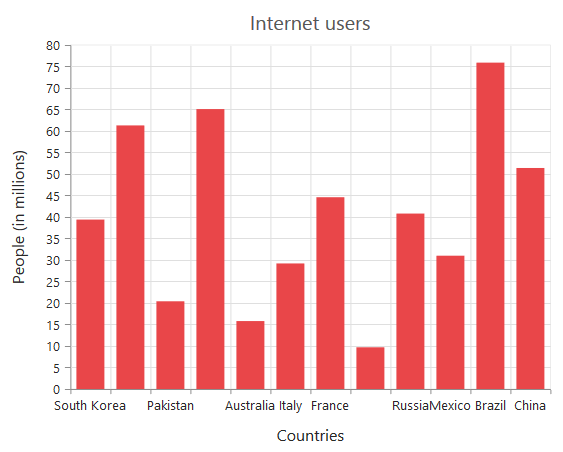The following screenshot displays the result, when the `labelIntersectAction` property is set as **multipleRows **.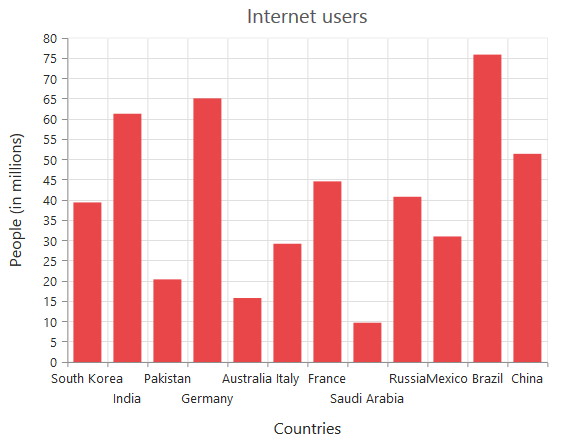The following screenshot displays the result, when the `labelIntersectAction` property is set as wrapByWord.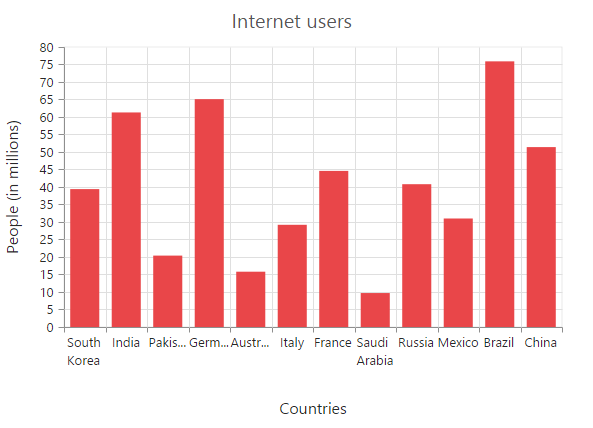## Multi-level Labels

Axis can be customized with multiple levels of labels using the [`multiLevelLabels`] property. These labels are placed based on the start and end range values and we can add any number of labels to an axis.

• javascript
• ``````"use strict";

var primaryXAxis=
{
multiLevelLabels: [
{
visible: true,
start: -0.5,
end: 2.5,
text: "Quater1"
}]
};
ReactDOM.render(
<EJ.Chart id="default_chart_sample_0"
primaryXAxis={primaryXAxis}
>

</EJ.Chart>,
document.getElementById('chart')
);``````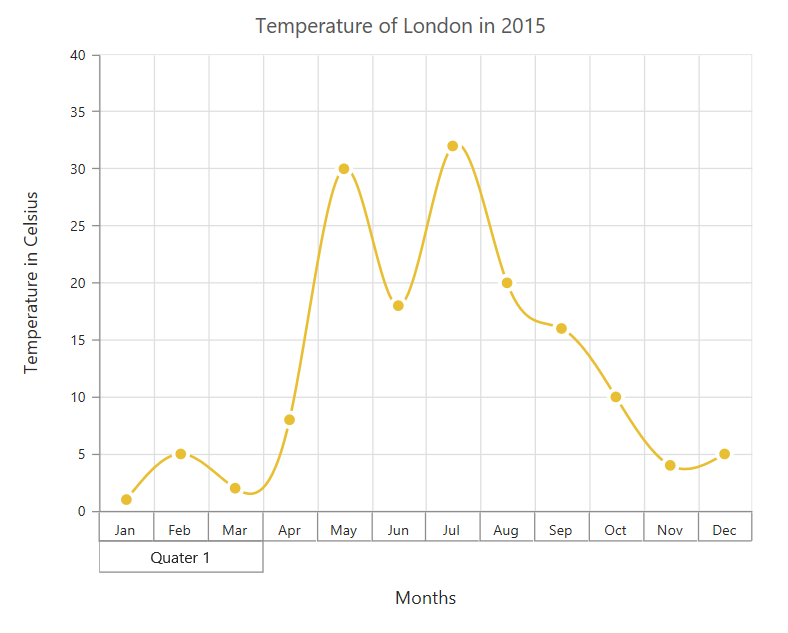### Customizing the multi-Level labels

The color, width and type of the border can be customized. The default border type is [`Rectangle`]. And the other supported border types are namely brace, curly brace, without top/bottom border and none.

• javascript
• ``````"use strict";
var primaryXAxis = {
multiLevelLabels: [
{
// customizing the border properties
border:{
type: "brace",
width: 2,
color: "black"
}
}]
}

ReactDOM.render(
<EJ.Chart id="default_chart_sample_0"
primaryXAxis={primaryXAxis}
>

</EJ.Chart>,
document.getElementById('chart')
);``````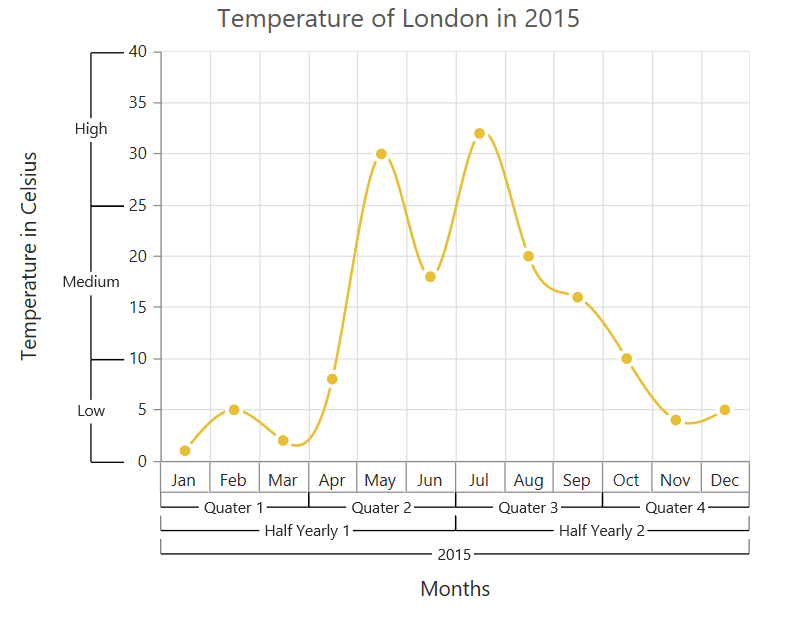The text of the labels can be customized using the [`text`] and [`font`] properties

• javascript
• ``````"use strict";
var primaryXAxis = {
multiLevelLabels: [
{
// customizing the text and font properties
text: "Year - 2015",
font:{
fontFamily: "Algerian",
size: "12px",
color: "black"
}
}]
}

ReactDOM.render(
<EJ.Chart id="default_chart_sample_0"
primaryXAxis={primaryXAxis}
>

</EJ.Chart>,
document.getElementById('chart')
);``````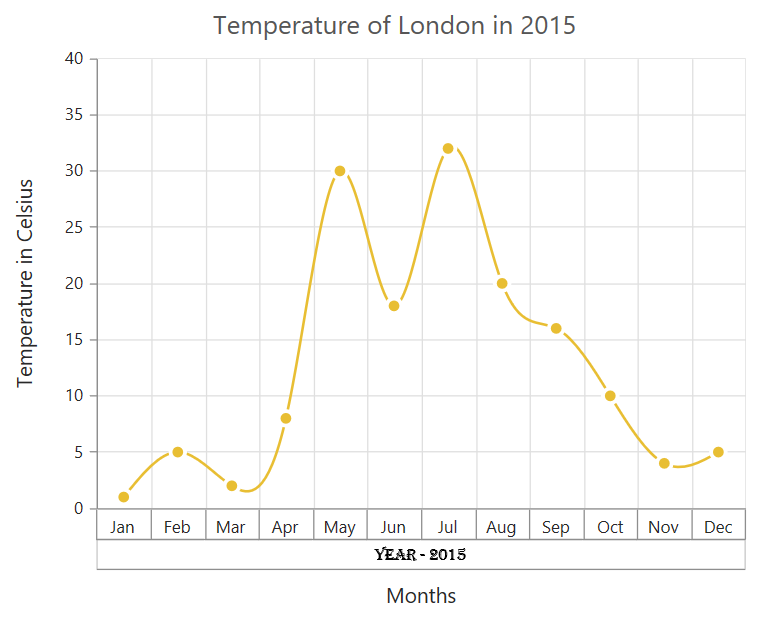You can change the alignment of the text to far, near and center position using the [`textAlignment`] property. By default, the text will be center aligned.

• javascript
• ``````"use strict";
var primaryXAxis = {
multiLevelLabels: [
{
// customizing the text alignment
textAlignment: "far",
}]
}

ReactDOM.render(
<EJ.Chart id="default_chart_sample_0"
primaryXAxis={primaryXAxis}
>

</EJ.Chart>,
document.getElementById('chart')
);``````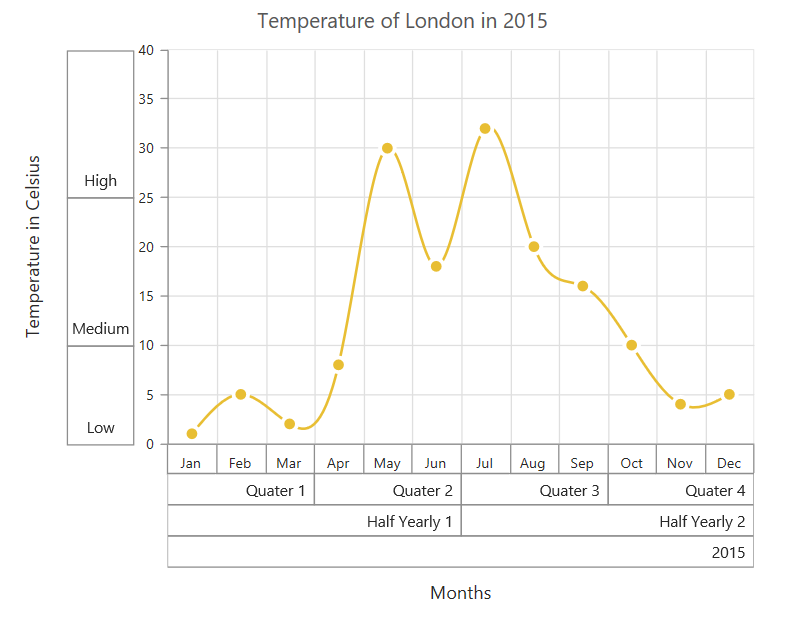You can trim, wrap or wrapAndTrim the text if it exceeds the maximum text width value using the property [`textOverflow`]

• javascript
• ``````"use strict";
var primaryXAxis = {
multiLevelLabels: [
{
// customizing the text overflow
textOverflow: "trim",
maximumtextWidth: 40
}]
}

ReactDOM.render(
<EJ.Chart id="default_chart_sample_0"
primaryXAxis={primaryXAxis}
>

</EJ.Chart>,
document.getElementById('chart')
);``````

The below screenshot shows the trimmed multi-level labels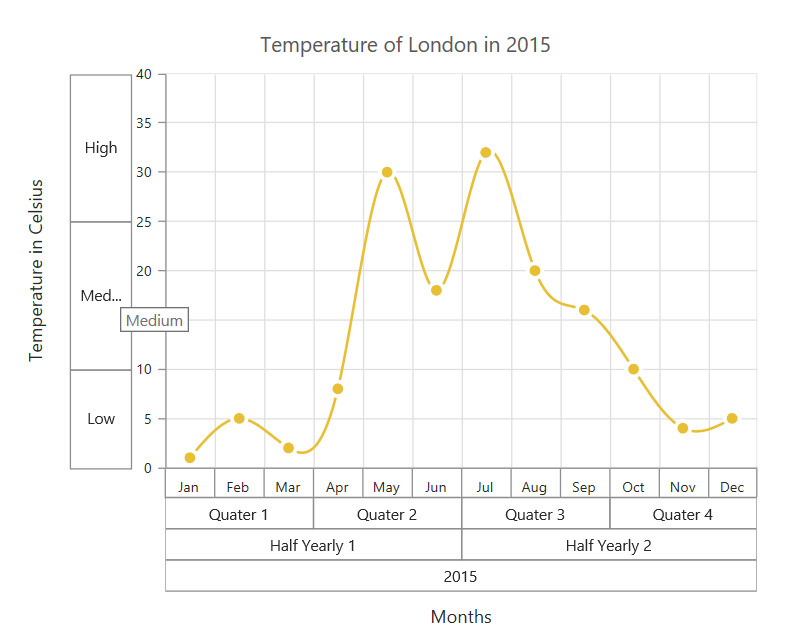And these labels can be placed in various rows using the [`level`] property.